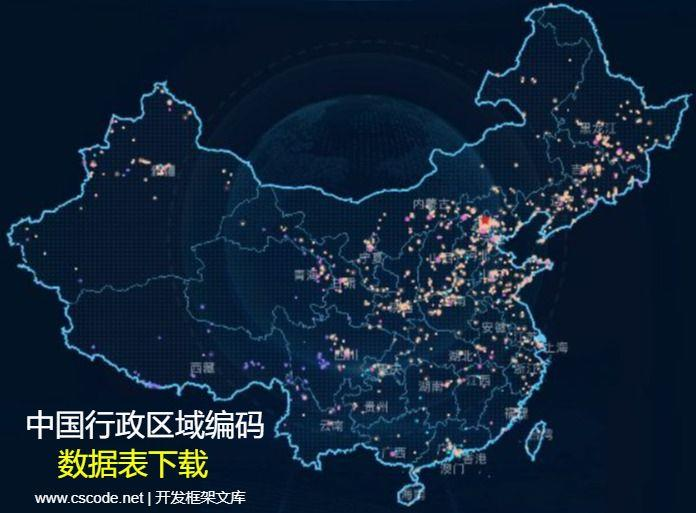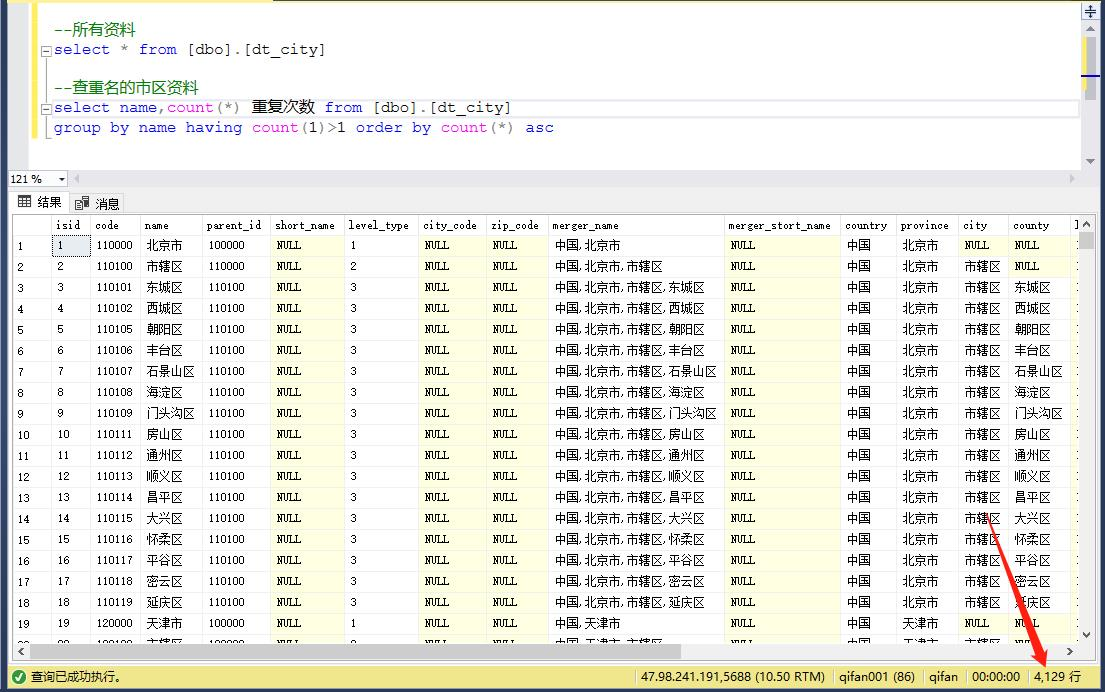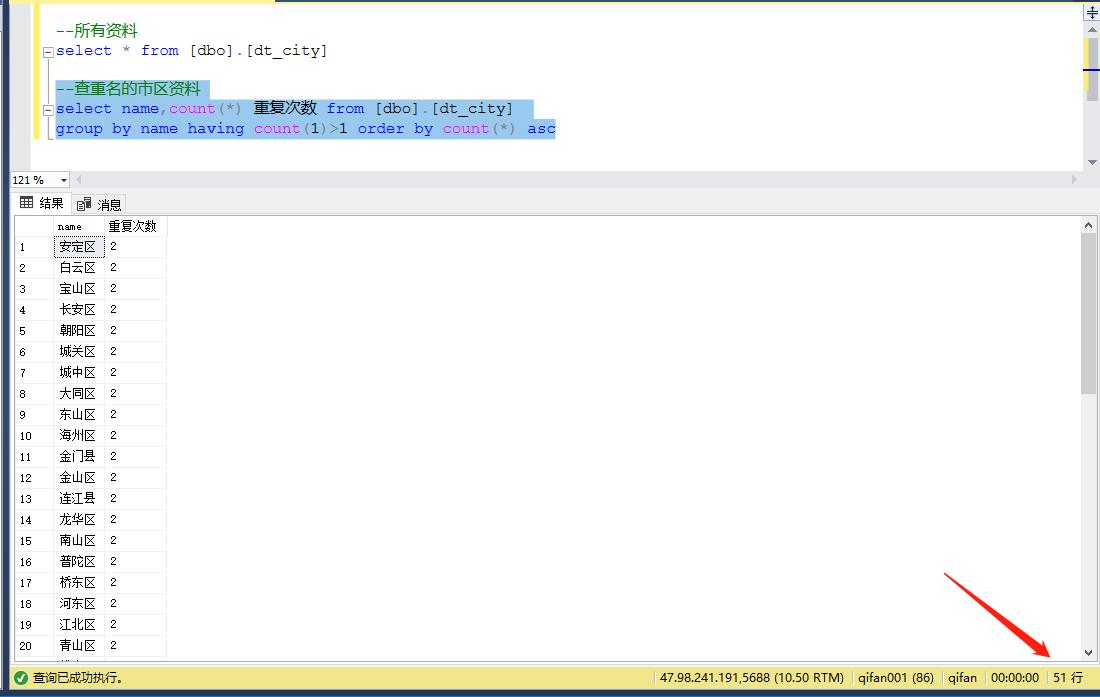dt_City表重复市区名称查询 | 中国行政区域编码数据表,省市区编号表|文档下载dt_City表重复市区名称查询 - 中国行政区域编码数据表,省市区编号表

## dt_city表

SQL 全选
``````--所有资料
select * from [dbo].[dt_city]``````## 区名重名的资料查询

SQL 全选
``````--查重名的市区资料
select name,count(*) from [dbo].[dt_city]
group by name having count(1)>1 order by count(*) asc``````• 安定区
• 白云区
• 宝山区
• 长安区
• 朝阳区
• 城关区
• 城中区
• 大同区
• 东山区
• 海州区
• 金门县
• 金山区
• 连江县
• 龙华区
• 南山区
• 普陀区
• 桥东区
• 河东区
• 江北区
• 青山区
• 松山区
• 太平区
• 泰山区
• 铁东区
• 通州区
• 西安区
• 西湖区
• 新市区
• 新兴区
• 信义区
• 永定区
• 向阳区
• 新城区
• 中西区
• 中正区
• 新华区
• 中山区
• 西区
• 铁西区
• 省直辖县级行政区划
• 郊区
• 桥西区
• 南区
• 和平区
• 大安区
• 城区
• 北区
• 鼓楼区
• 市中区
• 东区
• 市辖区